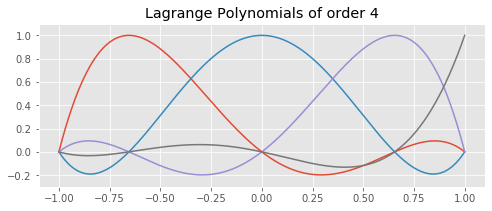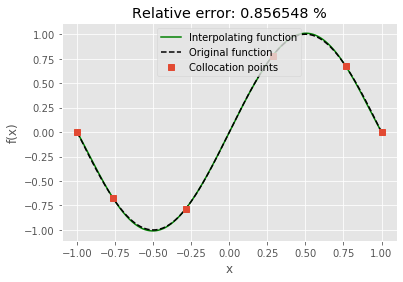Computational Seismology
Interpolation with Lagrange PolynomialsThis notebook is part of the supplementary material to Computational Seismology: A Practical Introduction, Oxford University Press, 2016.

##### Authors:¶

We can approximate an arbitrary function $f(x)$ using the interpolation with Lagrange polynomials $l_i$ at given collacation points $x_i$, i.e.

\begin{eqnarray*} f(x) = \sum f(x_i) \cdot l_i(x). \end{eqnarray*}

The Lagrange polynomials at $x$ are defined as follows:

$$\ell_i^{(N)} (x) \ := \ \prod_{k = 1, \ k \neq i}^{N+1} \frac{x - x_k}{x_i-x_k}, \qquad i = 1, 2, \dotsc , N + 1$$

They are implemented in Python with the following code:

In :
# Setup
%matplotlib inline

import numpy as np
import matplotlib.pyplot as plt
from gll import gll

# Prettier plots.
plt.style.use('ggplot')

In :
def lagrange2(N, i, x, xi):
"""
Function to calculate  Lagrange polynomial for order N
and polynomial i [0, N] at location x at given collacation points xi
(not necessarily the GLL-points)
"""
fac = 1
for j in range(-1, N):
if j != i:
fac = fac * ((x - xi[j + 1]) / (xi[i + 1] - xi[j + 1]))
return fac

N = 4
x = np.linspace(-1, 1, 1000)
xi, _ = gll(N)

plt.figure(figsize=(8, 3))
for _i in range(N):
plt.plot(x, lagrange2(N, _i, x, xi))
plt.ylim(-0.3, 1.1)
plt.title("Lagrange Polynomials of order %i" % N)
plt.show()## Exercises:¶

### 1. The GLL-points¶

• Use the gll() routine to determine the collocation points for a given order $N$ in the interval $[-1,1]$.
• Define an arbitrary function $f(x)$ and use the function lagrange(N,i,x) to get the $i$-th Lagrange polynomials of order N at the point x.
• Calculate the interpolating function to $f(x)$.
• Show that the interpolation is exact at the collocation points.
• Compare the original function $f(x)$ and the interpolating function on a finely spaced grid. Vary the order of the interpolating polynomials and calculate the error as a function of order.

### 2. Equidistant collocation points and the Runge function¶

In the first exercise we used the GLL-points that are not equidistant. Now use equidistant collacation points for the interpolation of your function $f$. Compare the two results.

Change the code for the Runge-function on the interval $[-5,5]$

$$f(x)=\frac{1}{(1+x^2)}$$

What do you notice when you decrease the order of your interpolation?

In :
# CHOOSE THE EXERCISE
exercise = 1

# Exercise 1
if exercise == 1:
# Initialize space in the interval [-1, 1]
nx = 1000
x = np.linspace(-1, 1, nx)

# CHANGE FUNCTION HERE. Currently a simple sine function.
f = np.sin(np.pi * x)

# Exercise 2
elif exercise == 2:
# Initialize space in the interval [-5, 5]
nx = 1000
x = np.linspace(-5, 5, nx)
# CHANGE FUNCTION HERE. Currently the Runge function.
f = 1/(1 + x ** 2)

# Get order of Lagrange polynomial
# Uncomment for interactive use.
# N = int(input(' Give polynomials degree (N): '))
N = 5

if exercise == 1:
# Get collocation points xi from gll routine
[xi, w] = gll(N)
fi = np.interp(xi, x, f)
elif exercise == 2:
xi = np.linspace(-5, 5, N+1)
fi = np.interp(xi, x, f)

# Initialize Lagrange polynomials on the defined grid
lp = np.zeros((N + 1, len(x)))
for i in range(0, len(x)):
for j in range(-1, N):
lp[j + 1, i] = lagrange2(N, j, x[i], xi)

# Calculate interpolating polynomials by multiplying
# Lagrange polynomials with function values at xi
s = x * 0
for j in range(0, N + 1):
s = s + lp[j, :] * fi[j]

error = np.sum((np.abs(f - s))) / np.sum(np.abs(f)) * 100

# Plot results
plt.figure()
plt.plot(x, s, 'k-', color='green', label='Interpolating function')
plt.plot(x, f, 'k--', label='Original function')
plt.plot(xi, fi, 's', label='Collocation points')
plt.title('Relative error: %g %%' % error)
plt.xlabel('x')
plt.ylabel('f(x)')

if exercise == 1:
plt.legend(loc="upper center")
elif exercise == 2:
plt.xlim(-5, 5)
plt.legend(loc=2)

plt.show()